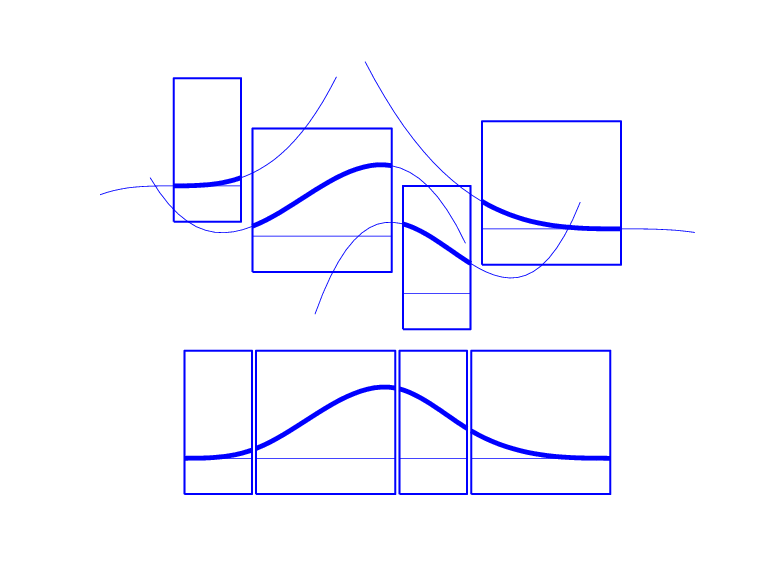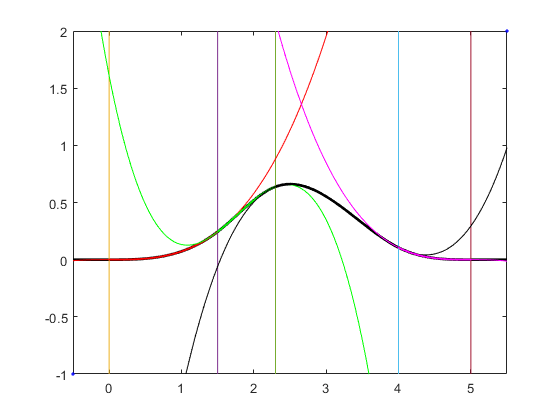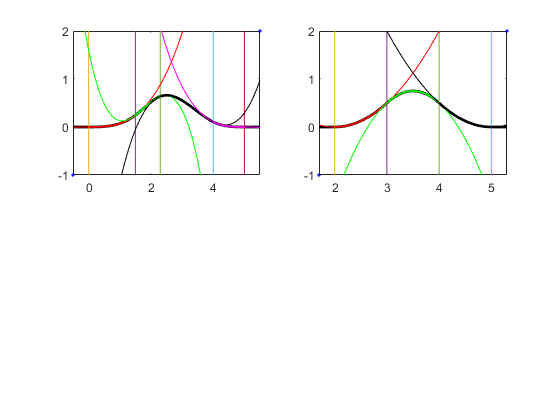# bspline

Plot B-spline and its polynomial pieces

## Syntax

``bspline(t)``
``bspline(t,window)``
``pp = bspline(t)``

## Description

example

````bspline(t)` plots the B-spline with knot sequence `t`, as well as the polynomial pieces of which it is composed. For more information about spline fitting, see About Splines in Curve Fitting Toolbox.```
````bspline(t,window)` plots the B-spline with knot sequence `t`, as well as the polynomial pieces of which it is composed, in the subplot window specified by `window`.```
````pp = bspline(t)` plots nothing and returns the ppform of the B-spline. ```

## Examples

collapse all

This example shows how to create a B-spline of order 4 using the `bspline` function.

Create a Knot Sequence and Plot the B-spline

This figure shows a B-spline of order 4 and the four cubic polynomials that composes it.To replicate this figure in MATLAB ®, first create a knot sequence. A knot sequence must be non-decreasing.

`t = [0 1.5 2.3 4 5];`

As you have defined five knots, the B-spline will be of order 4.

Then plot the B-spline with knot sequence `t`, as well as its polynomial pieces, by using the `bspline` function.

`bspline(t)`This B-spline consists of 4 polynomial pieces: the red, green, purple, and black curves in the plot, each of degree 3.

The vertical lines mark the knots `t` that you have previously defined.

The B-spline with knots t(i)≤····≤ t(i+k) is positive on the interval (t(i)..t(i+k)) and is zero outside that interval. It is piecewise-polynomial of order `k` with breaks at the sites t(i),...,t(i+k). These knots may coincide, and the precise multiplicity governs the smoothness with which the two polynomial pieces join there.

Plot Another B-spline in a New Subplot

Create a second knot sequence.

`t2=[2 3 4 5];`

Plot the B-spline with knot sequence `t2` in the same figure but in a different subplot.

```bspline(t,1) bspline(t2 ,2)```## Input Arguments

collapse all

Non-decreasing sequence of the knots of the B-spline, specified as a vector.

Data Types: `single` | `double`

Index of the subplot of a 2x2 window, specified as a scalar. This value must be less than 5.

Data Types: `single` | `double`

## Output Arguments

collapse all

Spline in ppform, returned as a structure with these fields. For more information on ppform, see The ppform

Form of the spline, returned as `pp`. `pp` indicates that the spline is given in piecewise polynomial form.

Knot positions of the spline, returned as a vector or as a cell array of vectors for multivariate data. Vectors contain strictly increasing elements that represent the start and end of each of the intervals over which the polynomial pieces are defined.

Coefficients of polynomials for each piece, returned as a matrix or as an array for multivariate data.

Number of polynomial pieces describing the spline, returned as a scalar or as a vector of numbers of pieces in each variable for multivariate data.

Order of the polynomial function describing each polynomial piece of the spline, returned as a scalar or as a vector containing the order in each variable for multivariate data.

Dimensionality of the target function, returned as a scalar.

## Version History

Introduced in R2006b